## Percentage

The importance of percentages is accentuated by the fact that there are a lot of questions related to the use of percentage in all the chapters of arithmetic. A closer look at the topic will evolve the result that at least 75% of the total calculations in any DI paper is formed on calculations on additions and percentages.
Percentage means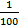• 100% = 100 x= 1
• To find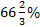of a number multiply it by 2/3
• To find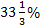of a number multiply it by 1/3
• To find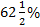of a number multiply it by 5/8
• To find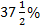of a number multiply it by 3/8
• To find 25% of a number multiply it by 1/4
• To find 20% of a number multiply it by 1/5
• To find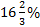of a number multiply it by 1/6
• To find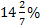of a number multiply it by 1/7
• To find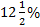of a number multiply it by 1/8
• To find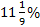of a number multiply it by 1/9
• To find 10% of a number multiply it by 1/10
• To find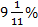of a number multiply it by 1/11
• To find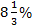of a number multiply it by 1/12
• To find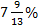of a number multiply it by 1/13
• To find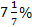of a number multiply it b 1/14
• To find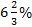of a number multiply it by 1/15
• To find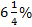of a number multiply it by 1/16
• Percentage increase =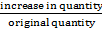x 100%
• Percentage decrease =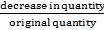x 100%
• To increase a number by x% multiply it by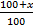• To decrease a number by x% multiply it by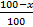• If A’s income is r% more than B’s income then B’s income is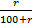x 100% less than A’s income.
• If A’s income is r% less than B’s income then B’s income isx 100% more than A’s income.
• If A’s income is 1/x more than B’s income, then B’s income is 1/(1 +x) less than A’s income.
• If A’s income is 1/x less than B’s income, then B’s income is 1/(x-1) more than A’s income
• If price of a commodity increases by x% then to keep the expenses same the consumption must be decreased by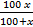%
• If price of a commodity decreases by x% then for same expense consumption can be increased by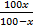%Want to Know More
Please fill in the details below:

Name

ltr
item
Clear CTET - Coaching Institute for CTET, DSSSB and KVS: dsssb-quants-percentage-theory-part1
dsssb-quants-percentage-theory-part1